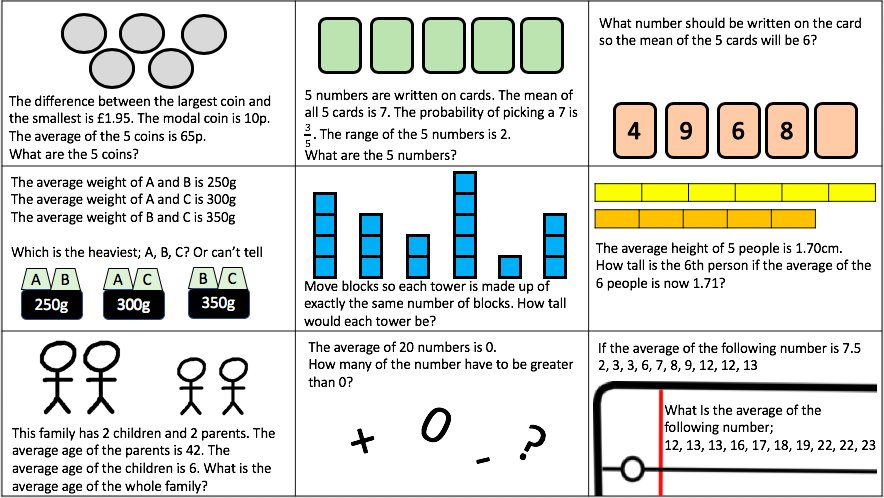# Points on a Line

Students thought about the problem for a little while before they discussed it in pairs. I invited students to share their ideas and this is what they came up with the suggestions below.I asked the students to explain the answers and they could use the grid on the visualiser to help. They came up and started marking on their points and realising one by one that the co-ordinates did not work. I then asked them to think a little bit longer about what the co-ordinates could be now they had a better idea of what we were/were not looking for

A students asked if they could mark a point on the line and say the co-ordinate and I said that was ok, so they quickly came up with (46.5, 11) so I asked if they could find anymore and they were trying to mark points between (45, 10) and (46.5, 11) but were not able to determine the co-ordinate.

# Averages and Range

I like to use the following starter when I start teaching Averages and Range. Students can use words such as Mode, Median, Mean and Range if they want to at this stage but it is nice for them to have a visual (actual blocks have been used where needed) and an example to refer to later in the lesson. If a student is struggling to calculate the mean, they could be reminded of how you break up the towers and rebuild them as towers of equal height.I find it easy to visualise all numbers as towers, even if i’m asked a question such as;

The scores on a recent Maths Test for a year 10 class are recorded and the mean is 24.4% for the class of 10 students.

Another Student joins the group, and the Mean is now 24%

What did this student score on the test?

I can imagine there are 10 towers of 24.4 blocks high. So there are 244 blocks in total. If the mean goes down to 24 blocks and there are 11 towers. We know there are 264 blocks. We can see there are 20 more blocks added so they scored 20%

Averages and Range 1

Averages and Range 2# Compound Measures

I have been looking at compound measure with year 11 this week and we have approached it using ratio tables. I like using ratio tables and i think it can make students think more logically about compound measures instead of substituting into formulae.

For example; I asked the class the following question…

I walk 2 kilometres and it takes me 15 minutes. At what speed do i travel at in km/h?

I had several students trying to draw a triangle and write S, D and T in the sections then try and divide 2 by 15.

Some tried to divide 2 by 0.25.

One student said ‘if you walk 2km in 15mins, you’ll walk 8km in an hour’

2 –> 15mins

… –> 1hour

This made sense to the class and we went from there. There was definitely a sense that the question and the process made more sense like this.Speed: Here are two slides of the same questions but one uses images the other doesn’t. Could be used as a match up or could be used to support students if they need it. Here’s the ppt: Compound Measures (Proportion)Density: Here are 3 slides that have the same questions in various forms.Pressure: And for Pressure…lesson 1 Pressure

lesson 2 Speed Distance Time

lesson 3 Density

UPDATE…Speed Proportion

# Compare

I like questions where students are asked to compare two options.  For example;I like to see how students answer these questions. The reason i have 30 is because i gave each question out to a students and they answered it in their book. I was interested in how they approached it and if they had more than one approach, I was also interested if the person next to them could find a simpler way. Here is the worksheet with all 30 questions. Feel free to use them how you wish. I give each student one each or stick one in each book when i take their books in. I then mark their responses.

30 Compare Questions